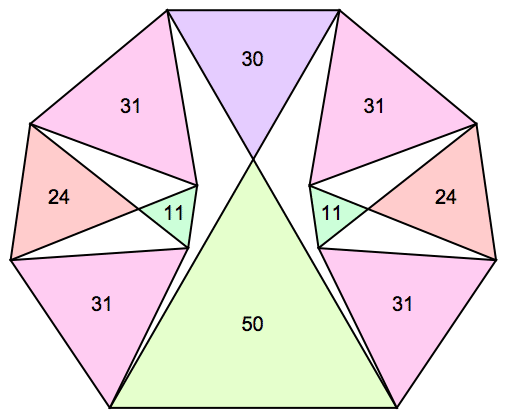1.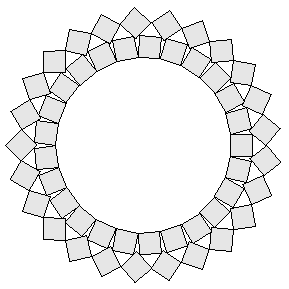2.

The other known solutions are shown below:

223772222 + 1036200452 = 11237853790239309
223772223 + 1036200453 = 1123785379023930900736173

2249002772 + 10364926272 = 1124897100420037858
2249002773 + 10364926273 = 1124897100420037858898449816

2237722202 + 10362004502 =1123785379023930900
2237722203 + 10362004503 =1123785379023930900736173000

421681727112 + 1087027311462 = 13594438548384283562837
421681727113 + 1087027311463 = 1359443854838428356283751827361567

704875606952 + 1117432313772 = 17455045971305066199154
704875606953 + 1117432313773 = 1745504597130506619915466723682008

1182808641702 + 10120437212252 = 1038222856499747505289525
1182808641703 + 10120437212253 = 1038222856499747505289525051686978625

9207263483102 + 10598298250782 = 1970976266597131517562184
9207263483103 + 10598298250783 = 1970976266597131517562184310094565552

3.

The answer is n(n–1)/2. This follows from the result that the expected number of rounds left at any stage is the sum of the pairwise products of the fortunes.

For example, if there are 3 players left with \$A, \$B, and \$C, then there are 6 equally likely outcomes of a player losing \$1 to another, and:

 6(AB+AC+BC – 1) = (A+1)(B–1)+(A+1)(C)+(B–1)(C) + (A+1)(B)+(A+1)(C–1)+(B)(C–1) + (A)(B+1)+(A)(C–1)+(B+1)(C–1) + (A)(B–1)+(A)(C+1)+(B–1)(C+1) + (A–1)(B+1)+(A–1)(C)+(B+1)(C) + (A–1)(B)+(A–1)(C+1)+(B)(C+1)

4.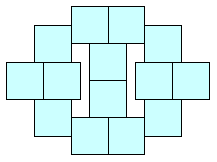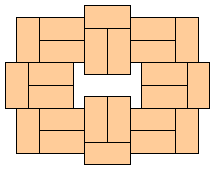5.

The tournament is as follows: A plays B a best-of-3, C plays D a best-of-3, and then the winners play. If at least one of the earlier best-of-3 matches takes only 2 games for a player to advance, then the winners play a best-of-3, other wise, they play a single game to decide the winner.

The probability that a best-of-3 match only lasts 2 games is (2/3)(2/3)+(1/3)(1/3) = 5/9. And the probability that the winner in this case is the better player is (4/9)/(5/9) = 4/5. This leaves 4/9 for the probability that a best-of-3 match lasts all 3 games, and in this case the probability the winner is the better player is 2/3. The chance that the better player wins a best-of-3 tournament in any way is (2/3)3 + 3(2/3)2(1/3) = 20/27.

So the probability that the best players wins the tournament described is (5/9)(5/9)(4/5)(20/27) + (5/9)(4/9)(4/5)(20/27) + (4/9)(5/9)(2/3)(20/27) + (4/9)(4/9)(2/3)(2/3) = 3536/6561 = 53.9%.

6.

 a)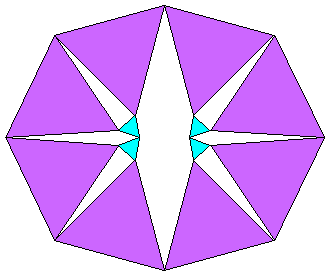b)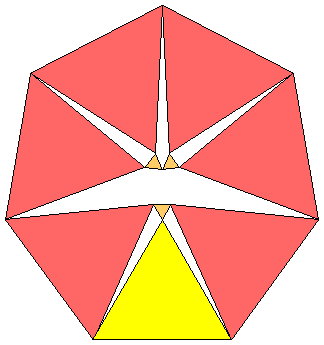c)